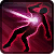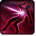# DE_Debug TeleportConditions

## Related effects, buffs and debuffs

• [0s] [not displayed]Effect #1

 Slot: Debuff Duration: 0 Sek. Tick rate: does not tick # occurrences: 0
• On Apply

Only when the following conditions are met:

• If a random chance of 25% is met

Perform the following actions:

• Execute Hydra Hook
- Event Name = (string) Teleport1
- Object Self = (int) 1
• Custom
- Param 2 = (string) SubEffect1
- Param 3 = (string)
- Param 4 = (string)
- Param 5 = (string)
- Param 6 = (string)
- Param 7 = (string)
- Param 8 = (string)
- Param 9 = (string)
- Param 1 = (int) 9420106349774076632
• On Apply

Only when the following conditions are met:

• <
• If a random chance of 33% is met
AND
• If previous subeffect did not meet all conditions
>

Perform the following actions:

• Execute Hydra Hook
- Event Name = (string) Teleport2
- Object Self = (int) 1
• Custom
- Param 2 = (string) SubEffect2
- Param 3 = (string)
- Param 4 = (string)
- Param 5 = (string)
- Param 6 = (string)
- Param 7 = (string)
- Param 8 = (string)
- Param 9 = (string)
- Param 1 = (int) 9420106349774076632
• On Apply

Only when the following conditions are met:

• <
• If a random chance of 50% is met
AND
• If previous subeffect did not meet all conditions
>

Perform the following actions:

• Execute Hydra Hook
- Event Name = (string) Teleport3
- Object Self = (int) 1
• Custom
- Param 2 = (string) SubEffect3
- Param 3 = (string)
- Param 4 = (string)
- Param 5 = (string)
- Param 6 = (string)
- Param 7 = (string)
- Param 8 = (string)
- Param 9 = (string)
- Param 1 = (int) 9420106349774076632
• On Apply

Only when the following conditions are met:

• If previous subeffect did not meet all conditions

Perform the following actions:

• Execute Hydra Hook
- Event Name = (string) Teleport4
- Object Self = (int) 1
• Custom
- Param 2 = (string) SubEffect4
- Param 3 = (string)
- Param 4 = (string)
- Param 5 = (string)
- Param 6 = (string)
- Param 7 = (string)
- Param 8 = (string)
- Param 9 = (string)
- Param 1 = (int) 9420106349774076632
• On Apply

Perform the following actions:

• Custom
- Param 2 = (string) Effect Fires Generally
- Param 3 = (string)
- Param 4 = (string)
- Param 5 = (string)
- Param 6 = (string)
- Param 7 = (string)
- Param 8 = (string)
- Param 9 = (string)
- Param 1 = (int) 9420106349774076632